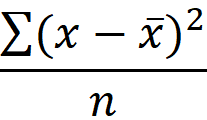The VARP function is a statistical function calculates and returns the variance of a population. Variance provides a general idea of how much data points are spread in a data set, and can be used to calculate the standard deviation or determine the risk of an investment. In this guide, we’re going to show you how to use the VARP function and also go over some tips and error handling methods.

Note that this function was replaced with the VAR.P function in Excel 2010. Although this function is still available for backwards compatibility, prefer using the VAR.P function instead.

## Supported versions

• Excel 2003 and newer versions

## VARP Function Syntax

VARP(number1,[number2],...)

## Arguments

 number1 The first number argument corresponding to entire population. [number2] Optional. Other number arguments up to 254 corresponding to entire population.

## Example

The VARP function accepts numeric values as its arguments. Any type of text or logical values will be ignored. You can use range references or static values just like in any other formula.

The function calculates the variance using the following function:• x: population mean (average)
• x ̅: element of the population
• n: population size

Here is the comparison of the two approaches:

=VARP(population)

=SUMPRODUCT(POWER(AVERAGE(population)-population,2))/COUNT(population)

The formulas using named range population (B5:B9).## VARP Function Tips

• Prefer using the VAR.P instead of the outdated VARP function.
• The function assumes that its arguments represent the entire population. If your data is a sample of the population, use VAR.S.
• The function ignores text and logical values like TRUE and FALSE. If you want to evaluate text values and FALSE as 0 and TRUE as 1, use VARPA.
• Empty cells are ignored.

## Issues

• Any error in the arguments causes the function to return an error.